# waynehu

## Professor, Department of Astronomy and Astrophysics University of Chicago

Group Contact CV SnapShots

## Damping

The photon-baryon fluid has slight imperfections corresponding to shear viscosity and heat conduction in the fluid [Weinberg, 1971]. These imperfections damp acoustic oscillations. To consider these effects, we now present the equations of motion of the system in their full form, including separate continuity and Euler equations for the baryons. Formally the continuity and Euler equations follow from the covariant conservation of the joint stress-energy tensor of the photon-baryon fluid. Because photon and baryon numbers are separately conserved, the continuity equations are unchanged,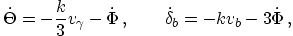(18)

where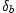and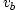are the density perturbation and fluid velocity of the baryons. The Euler equations contain qualitatively new terms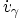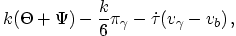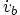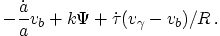(19)

For the baryons the first term on the right accounts for cosmological expansion, which makes momenta decay as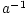. The third term on the right accounts for momentum exchange in the Thomson scattering between photons and electrons (protons are very tightly coupled to electrons via Coulomb scattering), with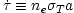the differential Thomson optical depth, and is compensated by its opposite in the photon Euler equation. These terms are the origin of heat conduction imperfections. If the medium is optically thick across a wavelength,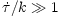and the photons and baryons cannot slip past each other. As it becomes optically thin, slippage dissipates the fluctuations.

In the photon Euler equation there is an extra force on the rhs due to anisotropic stress gradients or radiation viscosity in the fluid,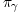. The anisotropic stress is directly proportional to the quadrupole moment of the photon temperature distribution. A quadrupole moment is established by gradients in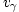as photons from say neighboring temperature crests meet at a trough (see Plate 3, inset). However it is destroyed by scattering. Thus, where the order unity constant can be derived from the Boltzmann equation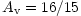[Kaiser, 1983]. Its evolution is shown in Figure 3. With the continuity Equation (7),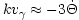and so viscosity takes the form of a damping term. The heat conduction term can be shown to have a similar effect by expanding the Euler equations in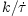. The final oscillator equation including both terms becomes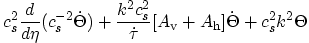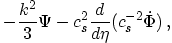(20)

where the heat conduction coefficient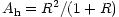. Thus we expect the inhomogeneities to be damped by a exponential factor of order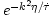(see Figure 3). The damping scale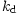is thus of order, corresponding to the geometric mean of the horizon and the mean free path. Damping can be thought of as the result of the random walk in the baryons that takes photons from hot regions into cold and vice-versa [Silk, 1968]. Detailed numerical integration of the equations of motion are required to track the rapid growth of the mean free path and damping length through recombination itself. These calculations show that the damping scale is of order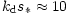leading to a substantial suppression of the oscillations beyond the third peak.

How does this suppression depend on the cosmological parameters? As the matter density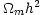increases, the horizon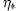decreases since the expansion rate goes up. Since the diffusion length is proportional to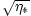, it too decreases as the matter density goes up but not as much as the angular diameter distance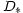which is also inversely proportional to the expansion rate. Thus, more matter translates into more damping at a fixed multipole moment; conversely, it corresponds to slightly less damping at a fixed peak number. The dependence on baryons is controlled by the mean free path which is in turn controlled by the free electron density: the increase in electron density due to an increase in the baryons is partially offset by a decrease in the ionization fraction due to recombination. The net result under the Saha approximation is that the damping length scales approximately as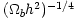. Accurate fitting formulae for this scale in terms of cosmological parameters can be found in [Hu & White, 1997c].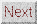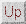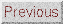Next: Polarization Up: ACOUSTIC PEAKS Previous: Radiation Driving
Wayne Hu 2001-10-15